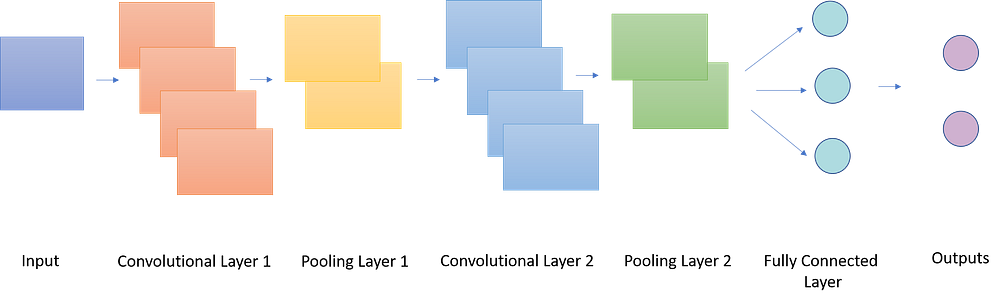# Basic Understanding of Convolutional Neural Network

Original article can be found here (source): Artificial Intelligence on Medium# Basic Understanding of Convolutional Neural Network

Today the world has made remarkable advancement in the field of Artificial Intelligence(AI). Researchers and mathematicians around the globe are probing different aspects by which they can create useful yet accurate applications, that can solve different human problems on its own without much human intervention. This article is focused on one branch of AI i.e. Convolutional Neural Network (CNN) & comprises of basic understanding of CNN.

Convolutional Neural Network is one of the branch of AI. CNN is a multi layered architecture that is specially designed for extracting and computing features (complex features) from data (particularly images) at each layer.

CNN is used for image recognition, object detection, image classification. Most useful applications of CNN includes :

1. Cancer Detection (e.g. Breast Cancer Detection)
2. Facial Recognition
3. Vehicle Detection

How CNN Works?

This section gives the bird eye view of how CNN algorithm basically works.

STEP 1:

CNN algorithm receives an image, it could be any image including cat, human, MRI scans etc. The image is in pixels form. If the image is in Grayscale or gray monochrome image then it would be comprising of single layer otherwise if it is RGB (Red-Blue-Green) images then three layers would be present i.e. one layer for each tone.

STEP 2:

The next step is to learn features using hidden layers (details will be discussed in later section). Feature detection may include edge detection, corner detection, ridge detection etc.

STEP 3:

After extracting useful features, the model will now be able to learn how to recognize an image. It can provide probability by detecting each image (test images).

Layers of CNN:

CNN model comprises of three types of hidden layers (as discussed in the previous section).

1. Convolutional Layer
2. Pooling Layer
3. Fully Connected Layer

I will briefly describe each layer in the next section.

1. Convolutional Layer:

Convolutional Layer is the layer where convolution process takes place. It uses set of filters (also known as kernels) to extract useful features from the images. A filter is used to detect features or patterns presented in the input image. There are many types of filters e.g. identity filters, edge detection filters, image sharpening filters.

The type of filter chosen will determine which features you want to extract or highlight particularly. E.g. if you wish to extract edges from the input image then use edge detection filters ( I am gonna write detail article about filters that would help you to grasp the concept). The depth of input image and filter should be the same. If your image is Grayscale image then filter should also be of one layer. The filter is then slided across the image to compute dot product between image pixels and filter values. There can be more than one convolutional layers. The output of Conv layer is a matrix that is called activation map.

Please note the more number of filters we have in the image, the more image features will be extracted and the network accuracy of detecting the new image will get high.

2. Pooling Layer:

Pooling layer is used to resize the image in order to decrease the computation power. This is basically the down sampling step. Pooling can be of two type:

1. Max Pooling: It will get the max value of pixel from the certain size of pixels matrix. E.g. if you are doing 2×2 max pooling than 2×2 pixel matrix from the convolved image will be chosen and the max value from that set of matrix will be pulled out.
2. Average Pooling: It will get the Average value of pixel from the certain size of pixels matrix. E.g. if you are doing 2×2 max pooling than 2×2 pixel matrix from the convolved image will be chosen and the Average value from that set of matrix will be pulled out.

In short, at every stride after convolution a max or avg value is selected.

3. Fully Connected Layer:

Fully connected layer is also sometimes referred as Dense Layer. It simply takes input from previous layers (Conv and Pooling Layer) and flattens them to one single vector. It basically contains the loss function. Then this output is forwarded to output layer that contains mostly Softmax function. Output layer performs classification and regression based on probabilities achieved through Softmax function.

Summary:

We pass an input image to convolutional layer where filters are applied to extract useful features from the images. The output of Conv layer is called activation map. Each filter is designated to extract specific feature. Pooling layer is then added to downsample image by reducing number of irrelevant parameters. There can be more than one Conv and pooling layer in CNN. The output layer is fully connected layer that works in a similar manner as in artificial neural network.

This was the basic theoretical overview of CNN architecture. I will give complete mathematical description in my next article. Hope you guys find this informative.